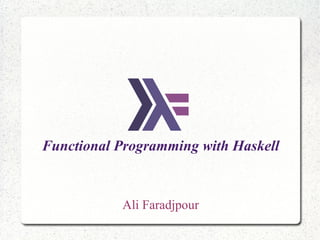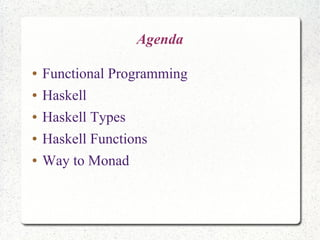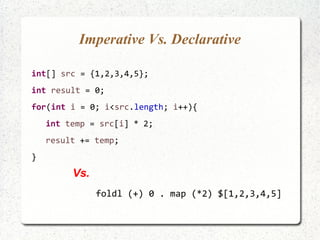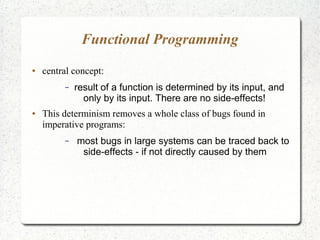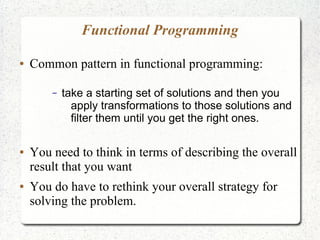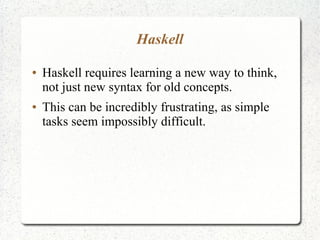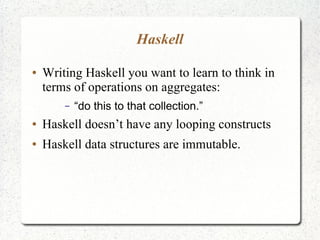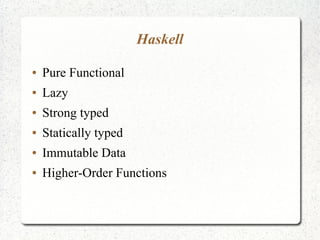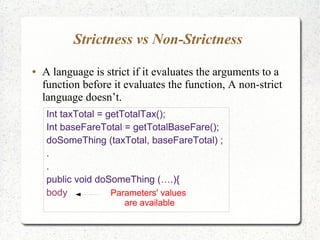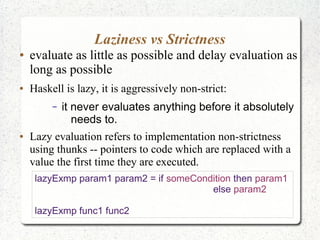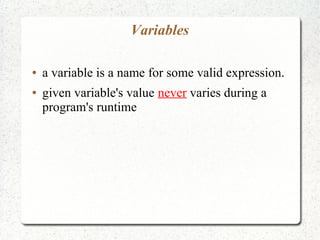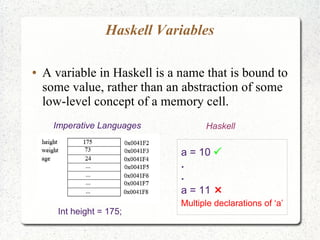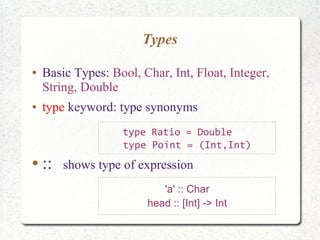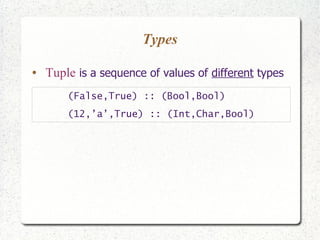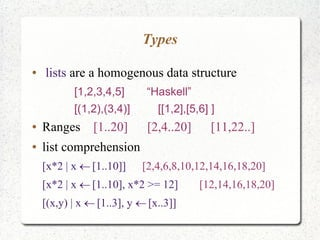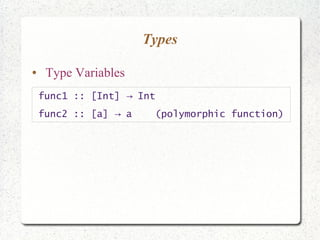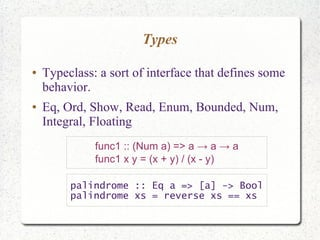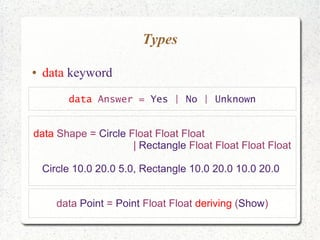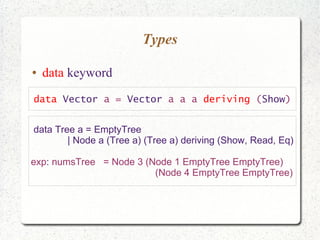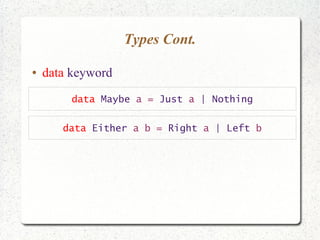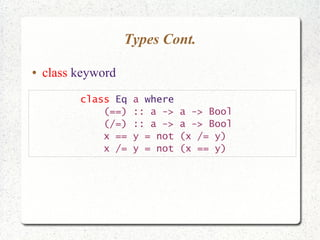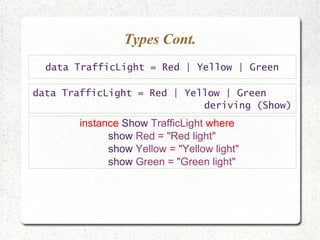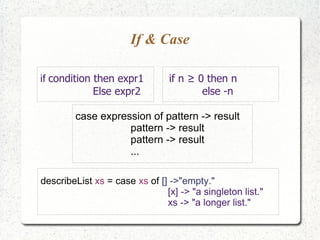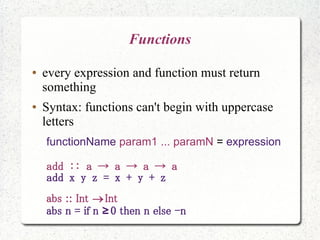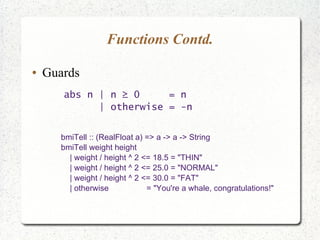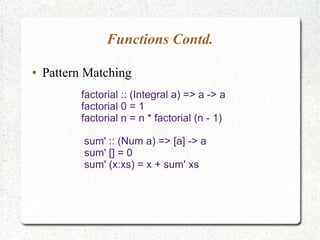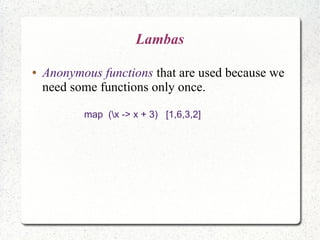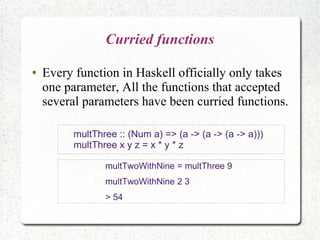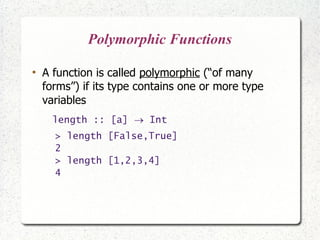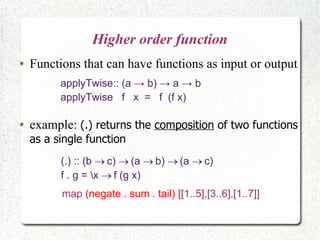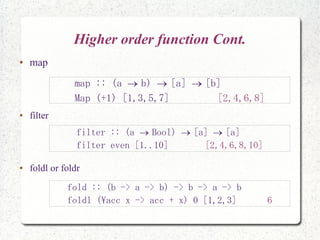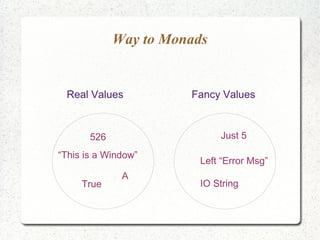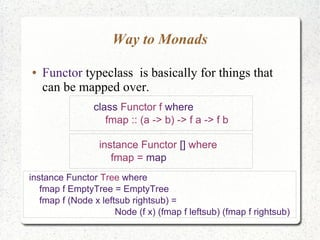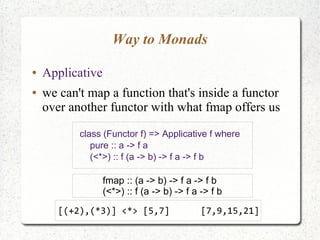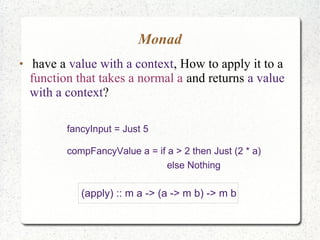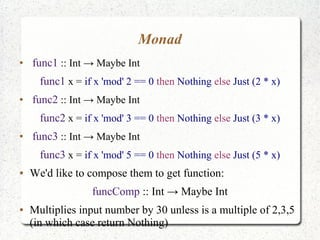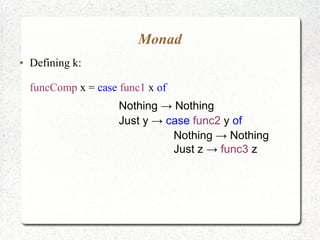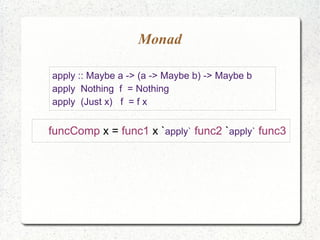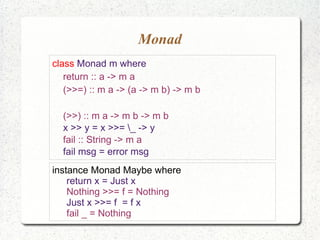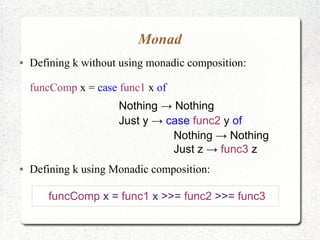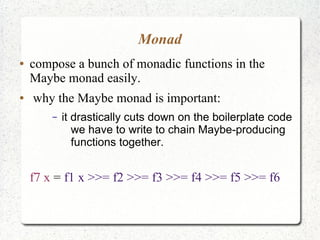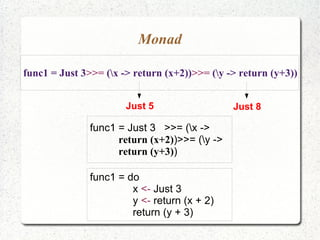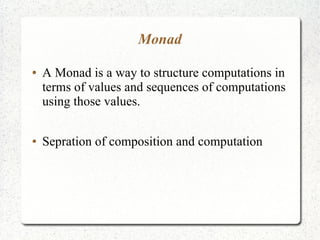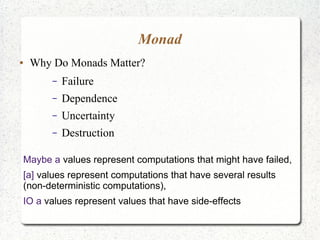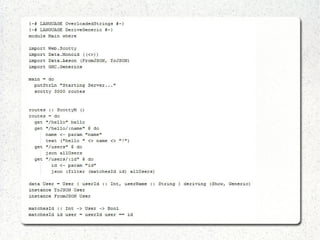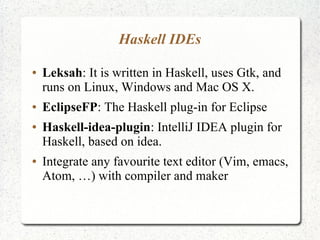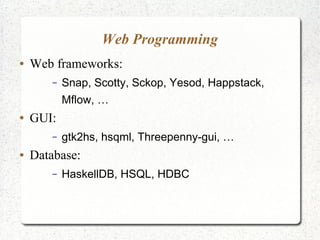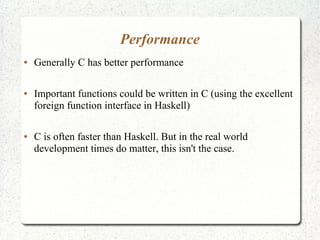1 of 49

3. Imperative Vs. Declarative int[] src = {1,2,3,4,5}; int result = 0; for(int i = 0; i<src.length; i++){ int temp = src[i] * 2; result += temp; } foldl (+) 0 . map (*2) \$[1,2,3,4,5] Vs.
4. Functional Programming ● central concept: – result of a function is determined by its input, and only by its input. There are no side-effects! ● This determinism removes a whole class of bugs found in imperative programs: – most bugs in large systems can be traced back to side-effects - if not directly caused by them
5. Functional Programming ● Common pattern in functional programming: – take a starting set of solutions and then you apply transformations to those solutions and filter them until you get the right ones. ● You need to think in terms of describing the overall result that you want ● You do have to rethink your overall strategy for solving the problem.
6. Haskell ● Haskell requires learning a new way to think, not just new syntax for old concepts. ● This can be incredibly frustrating, as simple tasks seem impossibly difficult.
7. Haskell ● Writing Haskell you want to learn to think in terms of operations on aggregates: – “do this to that collection.” ● Haskell doesn’t have any looping constructs ● Haskell data structures are immutable.
8. Haskell ● Pure Functional ● Lazy ● Strong typed ● Statically typed ● Immutable Data ● Higher-Order Functions
9. Strictness vs Non-Strictness ● A language is strict if it evaluates the arguments to a function before it evaluates the function, A non-strict language doesn’t. Int taxTotal = getTotalTax(); Int baseFareTotal = getTotalBaseFare(); doSomeThing (taxTotal, baseFareTotal) ; . . public void doSomeThing (….){ body Parameters' values are available
10. Laziness vs Strictness ● evaluate as little as possible and delay evaluation as long as possible ● Haskell is lazy, it is aggressively non-strict: – it never evaluates anything before it absolutely needs to. ● Lazy evaluation refers to implementation non-strictness using thunks -- pointers to code which are replaced with a value the first time they are executed. lazyExmp param1 param2 = if someCondition then param1 else param2 lazyExmp func1 func2
11. Variables ● a variable is a name for some valid expression. ● given variable's value never varies during a program's runtime
12. Haskell Variables ● A variable in Haskell is a name that is bound to some value, rather than an abstraction of some low-level concept of a memory cell. Imperative Languages Haskell a = 10  . . a = 11  Multiple declarations of ‘a’ Int height = 175;
13. Types ● Basic Types: Bool, Char, Int, Float, Integer, String, Double ● type keyword: type synonyms ● :: shows type of expression 'a' :: Char head :: [Int] -> Int type Ratio = Double type Point = (Int,Int)
14. Types ● Tuple is a sequence of values of different types (False,True) :: (Bool,Bool) (12,’a’,True) :: (Int,Char,Bool)
15. Types ● lists are a homogenous data structure [1,2,3,4,5] “Haskell” [(1,2),(3,4)] [[1,2],[5,6] ] ● Ranges [1..20] [2,4..20] [11,22..] ● list comprehension [x*2 | x [1..10]] [2,4,6,8,10,12,14,16,18,20] [x*2 | x [1..10], x*2 >= 12] [12,14,16,18,20] [(x,y) | x [1..3], y [x..3]] 
16. Types ● Type Variables func1 :: [Int] Int→ func2 :: [a] a (polymorphic function)→
17. Types ● Typeclass: a sort of interface that defines some behavior. ● Eq, Ord, Show, Read, Enum, Bounded, Num, Integral, Floating func1 :: (Num a) => a → a → a func1 x y = (x + y) / (x - y) palindrome :: Eq a => [a] -> Bool palindrome xs = reverse xs == xs
18. Types ● data keyword data Answer = Yes | No | Unknown data Shape = Circle Float Float Float | Rectangle Float Float Float Float Circle 10.0 20.0 5.0, Rectangle 10.0 20.0 10.0 20.0 data Point = Point Float Float deriving (Show)
19. Types ● data keyword data Vector a = Vector a a a deriving (Show) data Tree a = EmptyTree | Node a (Tree a) (Tree a) deriving (Show, Read, Eq) exp: numsTree = Node 3 (Node 1 EmptyTree EmptyTree) (Node 4 EmptyTree EmptyTree)
20. Types Cont. ● data keyword data Maybe a = Just a | Nothing data Either a b = Right a | Left b
21. Types Cont. ● class keyword class Eq a where (==) :: a -> a -> Bool (/=) :: a -> a -> Bool x == y = not (x /= y) x /= y = not (x == y)
22. Types Cont. data TrafficLight = Red | Yellow | Green instance Show TrafficLight where show Red = "Red light" show Yellow = "Yellow light" show Green = "Green light" data TrafficLight = Red | Yellow | Green deriving (Show)
23. If & Case if condition then expr1 Else expr2 if n ≥ 0 then n else -n case expression of pattern -> result pattern -> result pattern -> result ... describeList xs = case xs of [] ->"empty." [x] -> "a singleton list." xs -> "a longer list."
24. Functions ● every expression and function must return something ● Syntax: functions can't begin with uppercase letters functionName param1 ... paramN = expression add :: a → a → a → a add x y z = x + y + z abs :: Int Int abs n = if n 0 then n else -n≥
25. Functions Contd. ● Guards abs n | n ≥ 0 = n | otherwise = -n bmiTell :: (RealFloat a) => a -> a -> String bmiTell weight height | weight / height ^ 2 <= 18.5 = "THIN" | weight / height ^ 2 <= 25.0 = "NORMAL" | weight / height ^ 2 <= 30.0 = "FAT" | otherwise = "You're a whale, congratulations!"
26. Functions Contd. ● Pattern Matching factorial :: (Integral a) => a -> a factorial 0 = 1 factorial n = n * factorial (n - 1) sum' :: (Num a) => [a] -> a sum' [] = 0 sum' (x:xs) = x + sum' xs
27. Lambas ● Anonymous functions that are used because we need some functions only once. map (x -> x + 3) [1,6,3,2]
28. Curried functions ● Every function in Haskell officially only takes one parameter, All the functions that accepted several parameters have been curried functions. multTwoWithNine = multThree 9 multTwoWithNine 2 3 > 54 multThree :: (Num a) => (a -> (a -> (a -> a))) multThree x y z = x * y * z
29. Polymorphic Functions ● A function is called polymorphic (“of many forms”) if its type contains one or more type variables length :: [a]  Int > length [False,True] 2 > length [1,2,3,4] 4
30. Higher order function ● Functions that can have functions as input or output applyTwise:: (a → b) → a → b applyTwise f x = f (f x) (.) :: (b c) (a b) (a c)     f . g = x f (g x) ● example: (.) returns the composition of two functions as a single function map (negate . sum . tail) [[1..5],[3..6],[1..7]]
31. Higher order function Cont. ● map ● filter ● foldl or foldr map :: (a b) [a] [b]   Map (+1) [1,3,5,7] [2,4,6,8] filter :: (a Bool) [a] [a]   filter even [1..10] [2,4,6,8,10] fold :: (b -> a -> b) -> b -> a -> b foldl (acc x -> acc + x) 0 [1,2,3] 6
32. Way to Monads Real Values` Fancy Values 526 “This is a Window” True A Just 5 Left “Error Msg” IO String
33. Way to Monads ● Functor typeclass is basically for things that can be mapped over. class Functor f where fmap :: (a -> b) -> f a -> f b instance Functor [] where fmap = map instance Functor Tree where fmap f EmptyTree = EmptyTree fmap f (Node x leftsub rightsub) = Node (f x) (fmap f leftsub) (fmap f rightsub)
34. Way to Monads ● Applicative ● we can't map a function that's inside a functor over another functor with what fmap offers us class (Functor f) => Applicative f where pure :: a -> f a (<*>) :: f (a -> b) -> f a -> f b fmap :: (a -> b) -> f a -> f b (<*>) :: f (a -> b) -> f a -> f b [(+2),(*3)] <*> [5,7] [7,9,15,21]
35. Monad ● have a value with a context, How to apply it to a function that takes a normal a and returns a value with a context? fancyInput = Just 5 compFancyValue a = if a > 2 then Just (2 * a) else Nothing (apply) :: m a -> (a -> m b) -> m b
36. Monad ● func1 :: Int → Maybe Int func1 x = if x 'mod' 2 == 0 then Nothing else Just (2 * x) ● func2 :: Int → Maybe Int func2 x = if x 'mod' 3 == 0 then Nothing else Just (3 * x) ● func3 :: Int → Maybe Int func3 x = if x 'mod' 5 == 0 then Nothing else Just (5 * x) ● We'd like to compose them to get function: funcComp :: Int → Maybe Int ● Multiplies input number by 30 unless is a multiple of 2,3,5 (in which case return Nothing)
37. Monad ● Defining k: funcComp x = case func1 x of Nothing → Nothing Just y → case func2 y of Nothing → Nothing Just z → func3 z
38. Monad apply :: Maybe a -> (a -> Maybe b) -> Maybe b apply Nothing f = Nothing apply (Just x) f = f x funcComp x = func1 x `apply` func2 `apply` func3
39. Monad class Monad m where return :: a -> m a (>>=) :: m a -> (a -> m b) -> m b (>>) :: m a -> m b -> m b x >> y = x >>= _ -> y fail :: String -> m a fail msg = error msg instance Monad Maybe where return x = Just x Nothing >>= f = Nothing Just x >>= f = f x fail _ = Nothing
40. Monad ● Defining k without using monadic composition: funcComp x = case func1 x of Nothing → Nothing Just y → case func2 y of Nothing → Nothing Just z → func3 z ● Defining k using Monadic composition: funcComp x = func1 x >>= func2 >>= func3
41. Monad ● compose a bunch of monadic functions in the Maybe monad easily. ● why the Maybe monad is important: – it drastically cuts down on the boilerplate code we have to write to chain Maybe-producing functions together. f7 x = f1 x >>= f2 >>= f3 >>= f4 >>= f5 >>= f6
42. Monad func1 = Just 3>>= (x -> return (x+2))>>= (y -> return (y+3)) Just 5 Just 8 func1 = Just 3 >>= (x -> return (x+2))>>= (y -> return (y+3)) func1 = do x <- Just 3 y <- return (x + 2) return (y + 3)
43. Monad ● A Monad is a way to structure computations in terms of values and sequences of computations using those values. ● Sepration of composition and computation
44. Monad ● Why Do Monads Matter? – Failure – Dependence – Uncertainty – Destruction Maybe a values represent computations that might have failed, [a] values represent computations that have several results (non-deterministic computations), IO a values represent values that have side-effects
45. Haskell IDEs ● Leksah: It is written in Haskell, uses Gtk, and runs on Linux, Windows and Mac OS X. ● EclipseFP: The Haskell plug-in for Eclipse ● Haskell-idea-plugin: IntelliJ IDEA plugin for Haskell, based on idea. ● Integrate any favourite text editor (Vim, emacs, Atom, …) with compiler and maker
46. Web Programming ● Web frameworks: – Snap, Scotty, Sckop, Yesod, Happstack, Mflow, … ● GUI: – gtk2hs, hsqml, Threepenny-gui, … ● Database: – HaskellDB, HSQL, HDBC
47. Performance ● Generally C has better performance ● Important functions could be written in C (using the excellent foreign function interface in Haskell) ● C is often faster than Haskell. But in the real world development times do matter, this isn't the case.